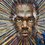# Do you want to Know Someone' s Birthday ??

I think you want to know someone' s birthday who wish not to tell you. So i ll tell you the simple trick for finding this. First let the month is x and date is y. Multiply 2 to x Add 5 to it Multiply 50 to it Add y to the answer. Result should be subtracted by 250. Thats it, for better understanding i ll give you an example.

If you re birthday is on January 1. 12=2 2+5=7 750=350 350+1=351

BIRTHDAY DATE= 351-250= 101 The last 2 digits are date and first two is month. if the number 1201, then 12= december, 01= date.

If you find it useful, please share it, if you like it, please like.Note by Anish Harsha
6 years, 7 months ago

This discussion board is a place to discuss our Daily Challenges and the math and science related to those challenges. Explanations are more than just a solution — they should explain the steps and thinking strategies that you used to obtain the solution. Comments should further the discussion of math and science.

When posting on Brilliant:

• Use the emojis to react to an explanation, whether you're congratulating a job well done , or just really confused .
• Ask specific questions about the challenge or the steps in somebody's explanation. Well-posed questions can add a lot to the discussion, but posting "I don't understand!" doesn't help anyone.
• Try to contribute something new to the discussion, whether it is an extension, generalization or other idea related to the challenge.
• Stay on topic — we're all here to learn more about math and science, not to hear about your favorite get-rich-quick scheme or current world events.

MarkdownAppears as
*italics* or _italics_ italics
**bold** or __bold__ bold
- bulleted- list
• bulleted
• list
1. numbered2. list
1. numbered
2. list
Note: you must add a full line of space before and after lists for them to show up correctly
paragraph 1paragraph 2

paragraph 1

paragraph 2

[example link](https://brilliant.org)example link
> This is a quote
This is a quote
    # I indented these lines
# 4 spaces, and now they show
# up as a code block.

print "hello world"
# I indented these lines
# 4 spaces, and now they show
# up as a code block.

print "hello world"
MathAppears as
Remember to wrap math in $$ ... $$ or $ ... $ to ensure proper formatting.
2 \times 3 $2 \times 3$
2^{34} $2^{34}$
a_{i-1} $a_{i-1}$
\frac{2}{3} $\frac{2}{3}$
\sqrt{2} $\sqrt{2}$
\sum_{i=1}^3 $\sum_{i=1}^3$
\sin \theta $\sin \theta$
\boxed{123} $\boxed{123}$

## Comments

Sort by:

Top Newest

How's this

- 6 years, 4 months ago

Log in to reply

Hi @Anish Harsha , I couldn't quite comprehend what you're trying to say . Can you help me out ? A new example could help .

Thanks $\ddot\smile$

- 6 years, 4 months ago

Log in to reply

You ask your friend the month, ( eg : January = 1, Febraury = 2, ..... ), then tell him to multiply with 2, then add 5, then multiply 50, then add the date to resulting number. Now ask him the final result, with that result subtract 250, then you'll get his birth month and date. ( eg : If the result number is 1201, then the thousandth and hundredth place is month = 12, the tenth and ones are date= 1, if there is no thousandth place then the month would be the hundredth place

- 6 years, 4 months ago

Log in to reply

Thanks @Anish Harsha , I understand now . :) Nice Algorithm .

Can you explain how did you derive this Algorithm ?

- 6 years, 4 months ago

Log in to reply

If we have to put date and month in the formula what do we find out???

- 6 years, 4 months ago

Log in to reply

this is not a logic....

- 6 years, 7 months ago

Log in to reply

hey guy look this solution will only come into action when u know the birthdate of the other person...... so how can trace his birthay by this formula??

- 6 years, 7 months ago

Log in to reply

ask him these questions and you ll get it

- 6 years, 7 months ago

Log in to reply

×

Problem Loading...

Note Loading...

Set Loading...